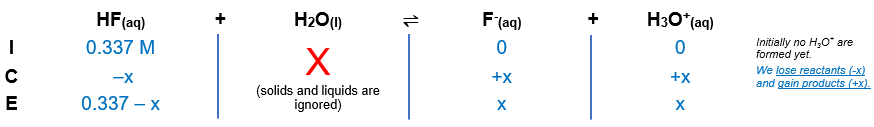# Problem: Find the percent ionization of a 0.337 M HF solution. The Ka for HF is 3.5 x 10-4. A) 4.7% B) 3.2% C) 1.2 x 10-2% D) 3.5 x 10-2%E) 1.1 %

###### FREE Expert Solution

HF(aq) + H2O(l)  F(aq) + H3O+(aq); Ka = 3.5 × 10–4

From this, we can construct an ICE table. Remember that liquids are ignored in the ICE table and Ka expression.The Ka expression for HF is:

$\overline{){{\mathbf{K}}}_{{\mathbf{a}}}{\mathbf{=}}\frac{\mathbf{products}}{\mathbf{reactants}}{\mathbf{=}}\frac{\mathbf{\left[}{\mathbf{H}}_{\mathbf{3}}{\mathbf{O}}^{\mathbf{+}}\mathbf{\right]}\mathbf{\left[}{\mathbf{F}}^{\mathbf{-}}\mathbf{\right]}}{\mathbf{\left[}\mathbf{HF}\mathbf{\right]}}}$

Plugging in the equilibrium concentrations from the ICE table into the Ka expression:

${\mathbf{K}}_{\mathbf{a}}\mathbf{=}\mathbf{3}\mathbf{.}\mathbf{5}\mathbf{×}{\mathbf{10}}^{\mathbf{-}\mathbf{4}}\mathbf{=}\frac{\mathbf{\left[}{\mathbf{H}}_{\mathbf{3}}{\mathbf{O}}^{\mathbf{+}}\mathbf{\right]}\mathbf{\left[}{\mathbf{F}}^{\mathbf{-}}\mathbf{\right]}}{\mathbf{\left[}\mathbf{HF}\mathbf{\right]}}$

${\mathbf{K}}_{\mathbf{a}}\mathbf{=}\mathbf{3}\mathbf{.}\mathbf{5}\mathbf{×}{\mathbf{10}}^{\mathbf{-}\mathbf{4}}\mathbf{=}\frac{\mathbf{\left(}\mathbf{x}\mathbf{\right)}\mathbf{\left(}\mathbf{x}\mathbf{\right)}}{\mathbf{0}\mathbf{.}\mathbf{337}\mathbf{-}\mathbf{x}}$

Now, we need to determine if we can remove (–x) from the equation. To do so, we need to determine the ratio of the initial concentration and Ka:

$\frac{\mathbf{\left[}\mathbf{HF}{\mathbf{\right]}}_{\mathbf{initial}}}{{\mathbf{K}}_{\mathbf{a}}}\mathbf{>}\mathbf{500}$

Since the ratio is greater than 500, we can remove (–x) from the equation.

The equation simplifies to:

79% (408 ratings)###### Problem Details

Find the percent ionization of a 0.337 M HF solution. The Ka for HF is 3.5 x 10-4

A) 4.7%

B) 3.2%

C) 1.2 x 10-2

D) 3.5 x 10-2%

E) 1.1 %

Frequently Asked Questions

What scientific concept do you need to know in order to solve this problem?

Our tutors have indicated that to solve this problem you will need to apply the Weak Acids concept. If you need more Weak Acids practice, you can also practice Weak Acids practice problems.

What professor is this problem relevant for?

Based on our data, we think this problem is relevant for Professor Haghishahir's class at Bluegrass Community and Technical College.